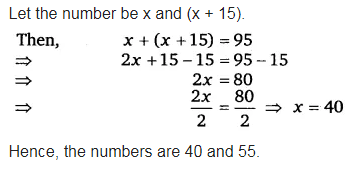# Sum of two numbers is 95. If one exceeds the other by 15, find the numbers

Sum of two numbers is 95. If one exceeds the other by 15, find the numbers.